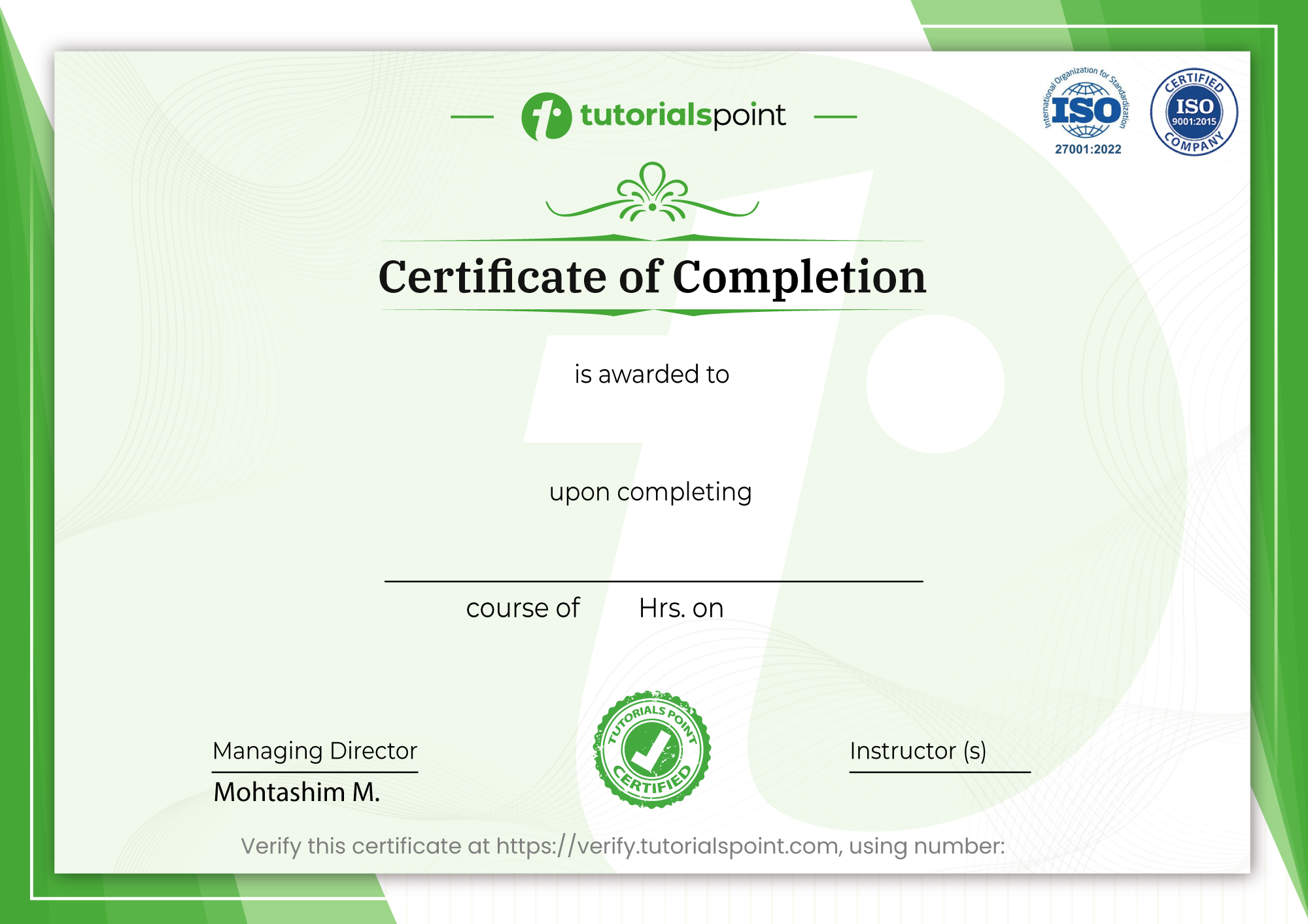Builds a strong foundation in Pre-Algebra & Algebra 1 for school & competitive exams

#### Course Description

This course is carefully designed to build a strong foundation in Algebra within a few hours. It will equip you with techniques required to tackle higher Algebra easily and is a stepping stone to your journey towards higher Mathematics.

The course consists of 84 video lectures spanning 11+ hours of on-demand videos that are divided into 17 sections. Each topic is explained extensively - by solving multiple questions along with the student during the lectures. The students are also provided and encouraged to solve practice questions & quizzes provided at the end of each topic.

Topics covered in the course:

• Basic Terminology of Algebra

• Finding the Numerical Value of Algebraic Expressions

• Addition and Subtraction of Algebraic Expressions

• Multiplication and Division of Algebraic Expressions

• Directed Numbers (Integers)

• Higher Indices (Exponent)

• Brackets in Algebra

• Linear Equations in One Variable

• Identities

• Change of Subject of Formula

• Linear Inequalities

• Factorization

• Simplification of Algebraic Fractions

• Coordinate Axis –Points and Line Graph

• Simultaneous Linear Equations in Two Variables

Here's what some students say about the course:

• "I just can't believe how fast I understand it. Awesome" - Emmet L Claret

• "Excellent content & superbly put together! The presentation is fantastic, the explanations are thorough, and the instructor's clarity and mastery over the subject are indeed commendable" - Apurva Jhamb

• "The course reminded everything that I left behind in earlier classes. And the course will definitely help me increase my speed in math. I am a student of class 12 and I'm grateful to see such a course which has cleared so many doubts which I had." - Shriyansh Patodia

• "This is very useful. It always helps in my studies" - Sapna Sankhala

• "Loved the content and explanation. Excellent!" - Sarthak Murarka

• "Very well explained" - Srishti Ladha

With this course, you'll also get:

• Complete support for any question, clarification, or difficulty you might face on the topic

• 30-day money-back guarantee

Feel free to contact me for any questions or clarifications you might have.

I look forward to seeing you on the course! :)

#### Who this course is for:

• Middle school, High school, or early college-level students
• Current IGCSE, CBSE & ICSE students (The course is specifically designed to cover these boards)
• GMAT, GRE, and MBA entrance exams students looking for a revision of Algebra fundamentals
• Anyone who wants to revise their Algebra

#### Goals

• Higher Indices - Laws of Indices (Exponent)

• Formula - Change the subject of the formula

• Rational Expressions - Simplification of Algebraic Fractions to its lowest form

• BODMAS - Adding and removing brackets in algebraic expressions

• Graphs - Coordinate Axis, Points, and Line Graph

• Linear equations in one variable and word problems

• Linear Inequalities

• Simultaneous linear equations - Graphical method, Substitution method, Equating coefficient & cross multiplication method

• Graphical method of solving simultaneous linear equations

• Word problems with the help of simultaneous linear equations

• Quadratic equations using the squaring complete method

• Equations reducible to quadratic equations

• Word problems of Quadratic equations

• Knowledge of the nature of roots of quadratic equations

• Zeros of polynomials α, β & γ

• Addition, Subtraction, Multiplication, and Division of Algebraic Expressions

• Remainder Theorem & Factor Theorem

• Directed Numbers (Integers)

• Finding the Numerical Value of Algebraic Expressions

• Factorization Techniques like common factors, regrouping, splitting the middle term, and using identities

• Algebraic Identities like ( a - b ) ² , ( a + b ) ³ , a ³ - b ³ , ( a + b + c ) ² etc

#### Prerequisites

• Knowledge of Mathematics till 5th grade

#### Curriculum

01:38
Preview
• Introduction - Important Instructions
01:24##### Feedbacks
• No Feedbacks Posted Yet..!##### This Course Includes
• 13 hours
• 116 Lectures
• 24 Resources
• 157 Quiz Questions
• Completion Certificate Sample Certificate
• Language English
• 30-Days Money Back Guarantee

#### Sample CertificateUse your certification to make a career change or to advance in your current career. Salaries are among the highest in the world.

We have 30 Million registered users and counting who have advanced their careers with us.

X

## Sample CertificateTalk to us